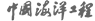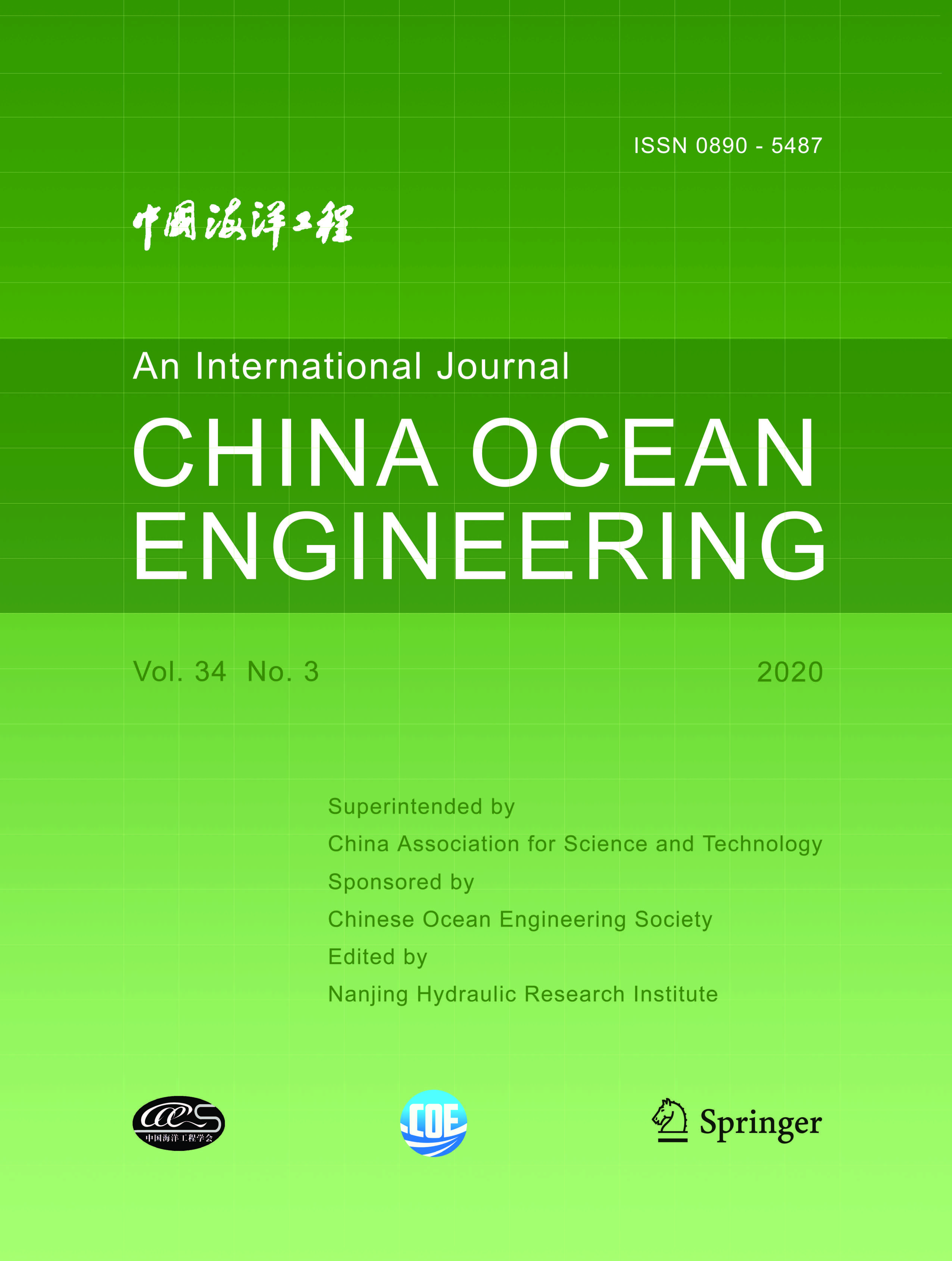ISSN  0890-5487 CN 32-1441/P

## 2003 Vol.(2)

Display Mode：          |

2003, (2): -.
[Abstract](415)
Abstract:
This paper proposes the stochastic analysis method of sea environment simulated by numerical models, such as wave height, current field, design sea levels and longshore sediment transport. Uncertainty and sensitivity analysis of input and output factors of numerical models, their long-term distribution and confidence intervals are described in this paper.
2003, (2): -.
[Abstract](328)
Abstract:
In this paper, a method is developed for determining the effective stiffness of the cracked component. The stiffness matrix of the cracked component is integrated into the global stiffness matrix of the finite element model of the global platform for the FE calculation of the structure in any environmental conditions. The stiffness matrix equation of the cracked component is derived by use of the finite variation principle and fracture mechanics. The equivalent parameters defining the element that simulates the cracked component are mathematically presented, and can be easily used for the FE calculation of large scale cracked structures together with any finite element program. The theories developed are validated by both lab tests and numerical calculations, and applied to the evaluation of crack effect on the strength of a fixed platform and a self-elevating drilling rig.
2003, (2): -.
[Abstract](335)
Abstract:
Model tests and numerical calculation of longshore currents and wave heights produced by irregular waves on two beaches with slopes of 1:100 and 1:40 are studied. The cross-shore distributions of longshore current velocities and wave heights are given and the influences of wave heights, wave periods, and beach slopes on longshore currents are discussed. The discussion is also made on the influences of different eddy viscosity coefficients on the numerical results of longshore current velocities.
2003, (2): -.
[Abstract](328)
Abstract:
Extensive 3-D model tests have been performed to study the effects of wave obliquity and multi-directionality on the wave loads acting on vertical breakwaters. The variation of horizontal and uplift forces acting on an unit length of a breakwater with wave direction, the longitudinal distribution of wave forces, as well as the longitudinal load reduction are analyzed. Some empirical formulae of the longitudinal distribution coefficient and the longitudinal load reduction factor are presented for practical use.
2003, (2): -.
[Abstract](316)
Abstract:
The design method of reinforcement of soft foundation with geotextile for the sea dike of the Zhapu Port is discussed in this paper. The prototype behaviours such as pore water pressure, settlement and so on were observed. The degree of consolidation is found out from observed pore water pressure and observed settlement respectively, then the strength increment of soil is calculated and compared with that obtained from vane shear tests. For the use of observed pore water pressure, the consolidation coefficient of soil is deduced approximately with a method named experimental exponential interpolation. The degree of consolidation of the ground is deduced theoretically from the dissipation of pore water pressure. Besides, the logarithmic curve and hyperbola are used to fit the observed time-settlement curve, and the degree of consolidation of soil is obtained according to the definition of the consolidation degree. After preliminary verification with observed prototype data, the method to reinforce the low dike with geotextile is considered to be simple and rational, and it can also reduce the construction cost.
2003, (2): -.
[Abstract](332)
Abstract:
Based on physical model tests, the rubble mound toe structure stability under the action of both regular and irregular waves is studied. Test results show that wave height and water depth at the toe structure are the most important factors affecting the stability of toe berm stone, and that irregular waves cause greater damage to the toe structure than regular waves. Analyses prove that the Gerding formula agrees better with our test results than the Meer formula. Tests on two different types of main armors also indicate that the shape and composition of the main armor have effect on the stability of the toe structure.
2003, (2): -.
[Abstract](327)
Abstract:
2003, (2): -.
[Abstract](324)
Abstract:
Considering that the thickness of a pontoon-type very large floating structure (VLFS) is very small in comparison with the length and width, VLFS can be modeled as a thin plate. In theory, the displacements and the membrane forces of a plate with large deflection are all the functions of the second-order differentials of the Ariy stress function. With these characteristics considered, the Ariy stress function of a floating free-free plate is calculated by setting the virtual values of three of the corner points. The finite difference method is chosen to solve the problem. When the Ariy stress function of the plate is obtained, the membrane forces can easily be calculated. Comparisons between the forces induced by the membrane forces and by the fluid are considered. It is shown that the membrane forces can not be neglected in many cases.
2003, (2): -.
[Abstract](322)
Abstract:
In this paper, the Artificial Neural Network (ANN) is used to study the wave forces on a semi-circular breakwater. The process of establishing the network model for a specific physical problem is presented. Networks with double implicit layers have been studied by numerical experiments. 117 sets of experimental data are used to train and test the ANN. According to the results of ANN simulation, this method is proved to have good precision compared with experimental and numerical results.
2003, (2): -.
[Abstract](312)
Abstract:
The measured spectrum for Storm 149 from North Alwyn is calculated by use of FFT (Fast Fourier Transformation) arithmetic and its statistical characteristics are used to determine the predicted spectrum in which the JONSWAP formula is adopted to review comparative object. It is shown that the agreement between measured and predicted spectrum is satisfactory and some corrections to the parameters for the JONSWAP formula are necessary.
2003, (2): -.
[Abstract](308)
Abstract:
In this paper, the control method for fixed offshore platforms using semi-active tuned liquid column damper (TLCD) is presented. The equation of motion for the platform-TLCD control system is given and the semi-active control strategy is established. A back propagation artificial neural network (ANN) is used to adjust the orifice opening of TLCD because of the nonlinear motion of liquid in TLCD. The effectiveness of the control method is verified by numerical examples.
2003, (2): -.
[Abstract](315)
Abstract:
In this paper, the neural network technology is combined with the fuzzy set theory to model the wave-induced ship motions in irregular seas. This combination makes possible the handling of a non-linear dynamic system with insufficient input information. The numerical results from the strip theory are used to train the networks and to demonstrate the validity of the proposed procedure.
2003, (2): -.
[Abstract](317)
Abstract:
2003, (2): -.
[Abstract](323)
Abstract:
The key problem in physical model tests with highly reflective structures is to prevent the multiple reflections between the reflective structures and the wave maker. An active absorption wave maker system is described and the representative frequency method for irregular waves is proposed in this paper. Physical model tests are conducted to verify the effectiveness of the proposed method.
2003, (2): -.
[Abstract](313)
Abstract:
Great attention has been paid to the development of very large floating structures. Owing to their extreme large size and great flexibility, the coupling between the structural deformation and fluid motion is significant. This is a typical problem of hydroelasticity. Efficient and accurate estimation of the hydroelastic response of very large floating structures in waves is very important for design. In this paper, the plate Green function and fluid Green function are combined to analyze the hydroelastic response of very large floating structures. The plate Green function here is a new one proposed by the authors and it satisfies all boundary conditions for free-free rectangular plates on elastic foundations. The results are compared with some experimental data. It is shown that the method proposed in this paper is efficient and accurate. Finally, various factors affecting the hydroelastic response of very large floating structures are also studied.

### Current Issue1. Editor-in-Chief:

2. Superintended by:

CHINA ASSOCIATION FOR SCIENCE AND TECHNOLOGY

Chinese Ocean Engineering Society （COES）

4. Edited by:

Nanjing Hydraulic Research InstituteAddress: 34 Hujuguan, Nanjing 210024, China Pos: 210024 Tel: 025-85829388 E-mail: coe@nhri.cn

Support by Beijing Renhe Information Technology Co. Ltd E-mail: info@rhhz.net